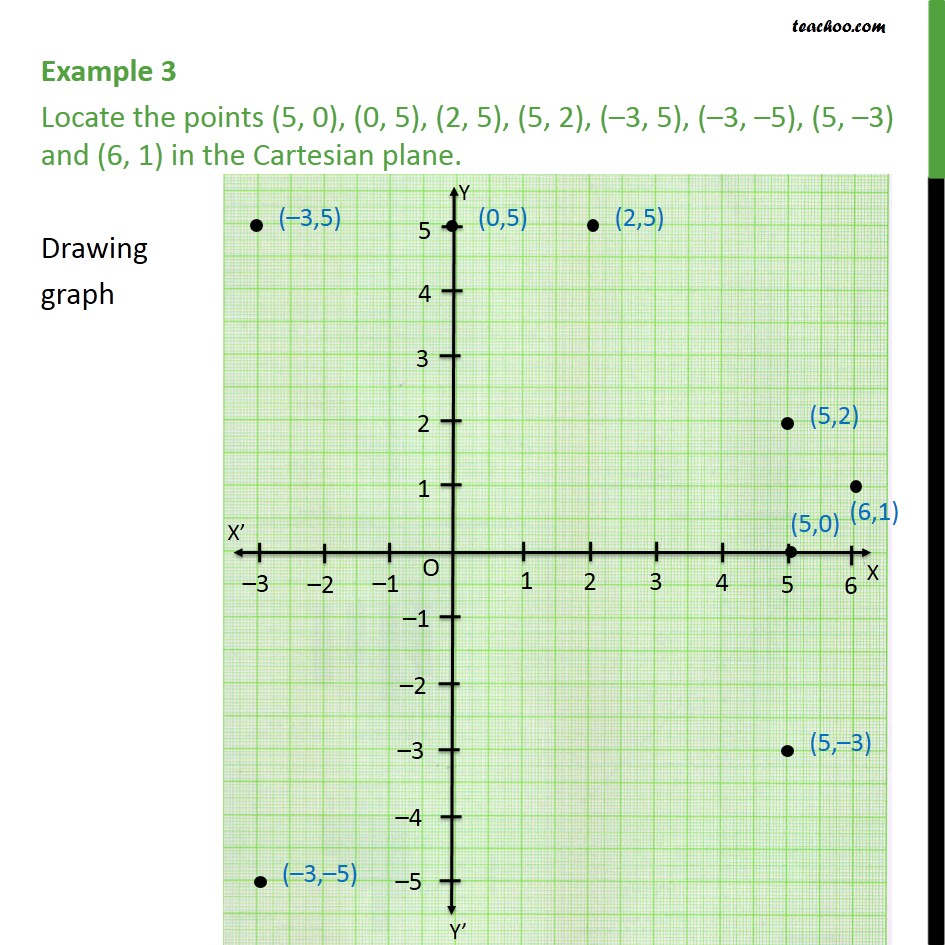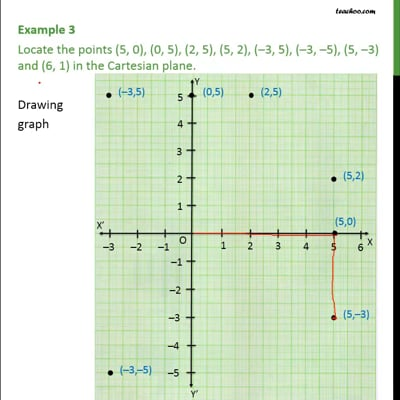Examples

Chapter 3 Class 9 Coordinate Geometry
Serial order wiseThis video is only available for Teachoo black users

Maths Crash Course - Live lectures + all videos + Real time Doubt solving!

### Transcript

Example 3 Locate the points (5, 0), (0, 5), (2, 5), (5, 2), (–3, 5), (–3, –5), (5, –3) and (6, 1) in the Cartesian plane. We follow these steps to mark points 1. Drawing x and y axis 2. Now, in all points, maximum value of x is 6, and minimum is -3. So, we draw x-axis from -3 to 6 3. Also, in all points, maximum value of y is 5, and minimum is -3. So, we draw y-axis from -3 to 5 4. Let's mark points one by one 5. For (5, 0), we go to 5 in x-axis. Now, since y is 0. We mark that point. 6. Similarly, we can plot all points